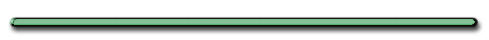HomeRelative Velocity   River with current. Label upstream and downstreamA upstream , B downstream     Ex 1) A boat's speed in still water is 1.85 m/s. If the boat is to travel directly across a river whose current is 1.20 m/s, at what upstream angle must the boat head?             sinӨ = [1.20 m/s]/[1.85 m/s]   Ө = 40.5° upstream     Crossing a River   When a boat crosses a river, which component(s) are affected. Vx? Vy?   Only Vx changed when boat crosses river     Vr = Velocity of river Vbx = Boat's x velocity Vby = Boat's y velocity Find Vx'   Vx' =                                   Vx' =                          Vx'=Path B Vx' = Vr Path A Vx' = Vbx - Vr Path C Vx' = Vbx + Vr   Downstream or straight across Vx increased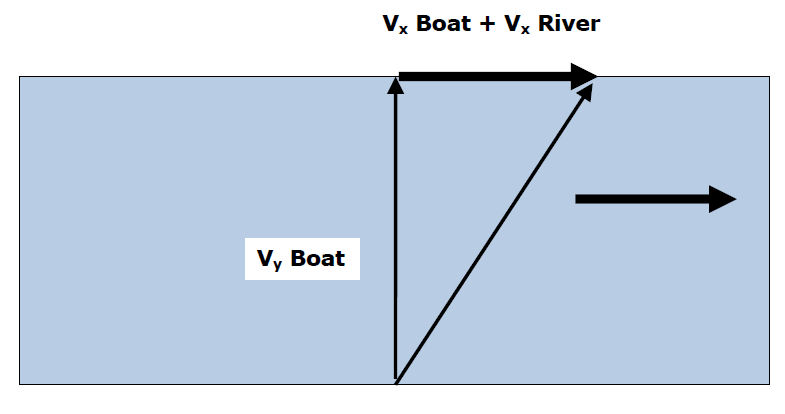Upstream Vx decreased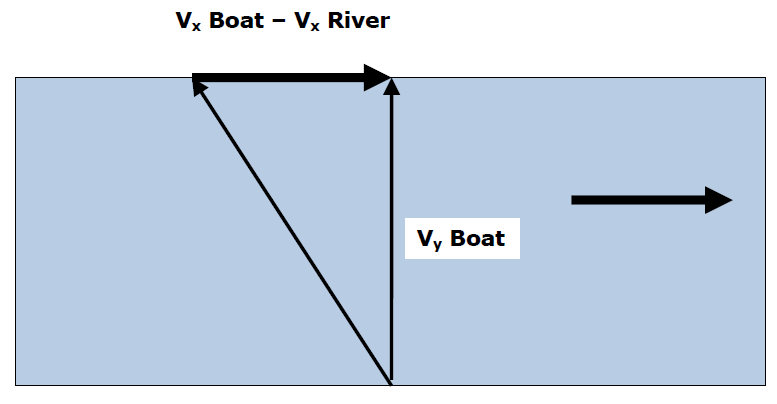Vx' = Vboat +/-  Vriver Vy = Vyboat V'2 = Vx'2 + Vy2   dx , dy?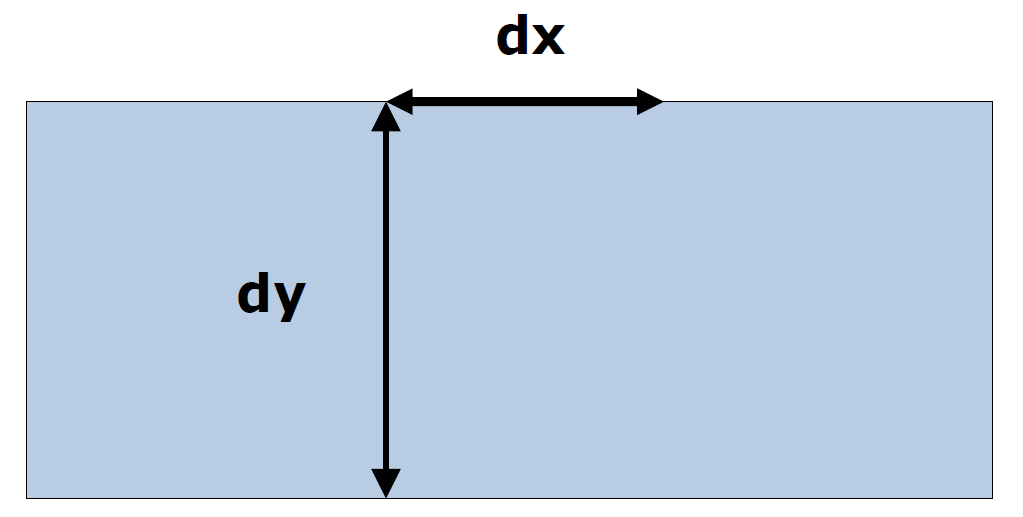dy = Vyt dx = (Vboat +- Vriver)tEx 2) A boat's speed in still water is of 10. m/s. The boat moves downstream across the river at an angle of 37 degrees from the shoreline and reaches opposite shore at point B. If the velocity of the river is 3.0 m/s, find the time of the trip and distance between A and B. The width of the river is 36 m.           dx = Vxt       Finding t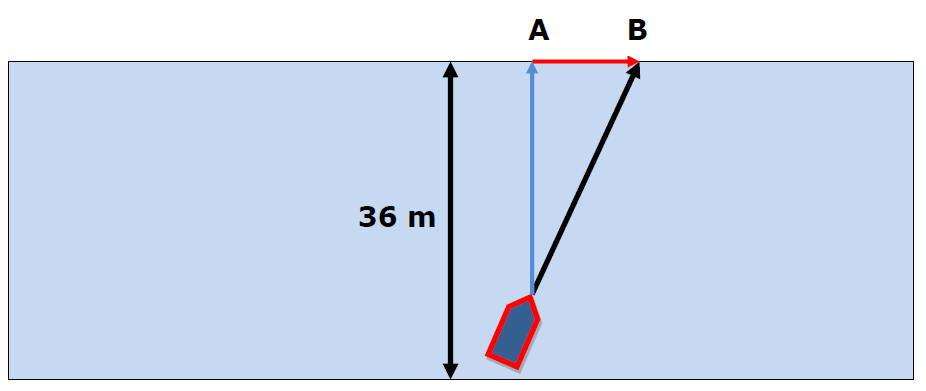dy = 36m   dy = Vyt = VsinӨt = 36 m36 m = 10 m/s[sin37]t   t = 6 sec    dx = Vxt     Finding Vx Vx = Vx boat + Vx river   Vx of Boat   Vx = 10 m/s[cos37] = 8 m/s Vx River = 3 m/s   Vx = 11 m/s   t = 6 sec    dx = Vxt dx = 66 m©Tony Mangiacapre., - All Rights Reserved [Home] Established 1995 Use any material on this site (w/ attribution)